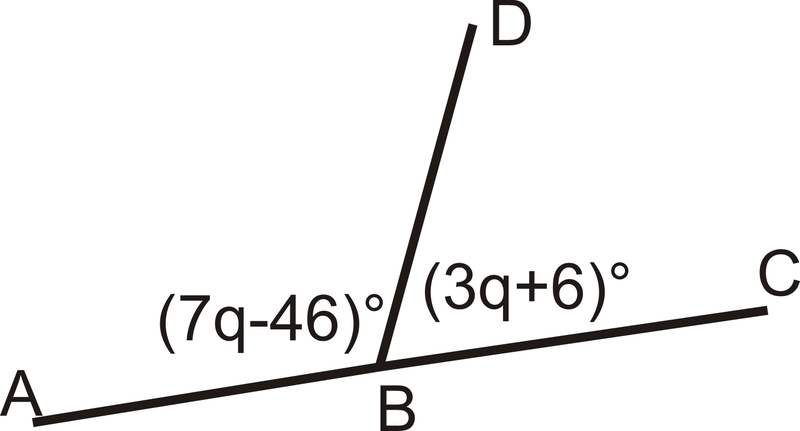# 1.15: Supplementary Angles

•• Contributed by CK12
• CK12

Two angles that add to 180 degrees and when adjacent form a straight line.

## Linear Pairs

Two angles are adjacent if they have the same vertex, share a side, and do not overlap. $$\angle PSQ$$ and $$\angle QSR$$ are adjacent.Figure $$\PageIndex{1}$$

A linear pair is two angles that are adjacent and whose non-common sides form a straight line. If two angles are a linear pair, then they are supplementary (add up to $$180^{\circ}$$). $$\angle PSQ$$ and $$\angle QSR$$ are a linear pair.Figure $$\PageIndex{2}$$

What if you were given two angles of unknown size and were told they form a linear pair? How would you determine their angle measures?

For Example $$\PageIndex{1}$$ and $$\PageIndex{2}$$, use the diagram below. Note that $$\overline{NK} \perp \overleftrightarrow{IL}$$.Figure $$\PageIndex{3}$$

Example $$\PageIndex{1}$$

Name one linear pair of angles.

Solution

$$\angle MNL$$ and $$\angle LNJ$$

Example $$\PageIndex{2}$$

What is $$m \angle INL$$?

Solution

$$180^{\circ}$$

Example $$\PageIndex{3}$$

What is the measure of each angle?Figure $$\PageIndex{4}$$

Solution

These two angles are a linear pair, so they add up to $$180^{\circ}$$.

$$(7q−46)^{\circ}+(3q+6)^{\circ}=180^{\circ}$$

$$10q−40^{\circ}=220^{\circ}$$

$$10q=180^{\circ}$$

$$q=22^{\circ}$$

Plug in q to get the measure of each angle.

$$m \angle ABD=7(22^{\circ})−46^{\circ}=108^{\circ}$$

$$m \angle DBC=180^{\circ}−108^{\circ}=72^{\circ}$$

Example $$\PageIndex{4}$$

Are $$\angle CDA$$ and $$\angle DAB$$ a linear pair? Are they supplementary?Figure $$\PageIndex{5}$$

Solution

The two angles are not a linear pair because they do not have the same vertex. They are supplementary because they add up to $$180^{\circ}: 120^{\circ}+60^{\circ}=180^{\circ}$$.

Example $$\PageIndex{5}$$

Find the measure of an angle that forms a linear pair with $$\angle MRS$$ if $$m \angle MRS$$ is $$150^{\circ}$$.

Solution

Because linear pairs have to add up to $$180^{\circ}$$, the other angle must be $$180^{\circ}−150^{\circ}=30^{\circ}$$.

## Review

For 1-5, determine if the statement is true or false.

1. Linear pairs are congruent.
2. Adjacent angles share a vertex.
4. Linear pairs are supplementary.
5. Supplementary angles form linear pairs.

For exercise 6, find the value of $$x$$.

1.Figure $$\PageIndex{6}$$

Find the measure of an angle that forms a linear pair with $$\angle MRS$$ if $$m \angle MRS$$ is:

1. $$61^{\circ}$$
2. $$23^{\circ}$$
3. $$114^{\circ}$$
4. $$7^{\circ}$$
5. $$179^{\circ}$$
6. $$z^{\circ}$$

## Vocabulary

Term Definition
Adjacent Angles Two angles are adjacent if they share aside and vertex. The word 'adjacent' means 'beside' or 'next-to'.
linear pair Two angles form a linear pair if they are supplementary and adjacent.
Diagram A diagram is a drawing used to represent a mathematical problem.

Interactive Element

Video: Complementary, Supplementary, and Vertical Angles

Activities: Supplementary Angles Discussion Questions

Study Aids: Angles Study Guide

Practice: Supplementary Angles

Real World: Supplementary Angles